# GRE Subject Test: Chemistry : Real Gases

## Example Questions

### Example Question #1 : Gases

Which of the following assumptions is not made by the ideal gas law?

The molecules move randomly

The van der Waals forces are negligible

The size of the molecules is much smaller than the container

The intermolecular interactions follow the Coulomb model of electric repulsion

The molecules obey Newton's laws of motion at all times

The intermolecular interactions follow the Coulomb model of electric repulsion

Explanation:

Under the ideal gas law, we assume that the interactions between the molecules are very brief and that the forces involved are negligible. The assumption that the molecules obey Coulomb's law when interacting with each other is not necessary; rather, an ideal gas must disregard Coulomb's law.

The ideal gas law assumes only Newtonian mechanics, disregarding any intermolecular or electromagnetic forces.

### Example Question #1 : Gases

Consider a real gas with a constant amount and a constant pressure. It has a temperature of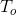and a volume of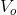. If you double the temperature, what will happen to the volume?

The volume will become greater than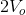The volume will become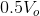The volume will becomeThe volume will become less thanThe volume will become less thanExplanation:

This question can be solved using either Charles's law or the ideal gas law (converted into the combined gas law).

Charles's Law: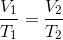Ideal Gas Law: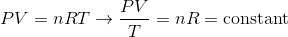The question states that the pressure and moles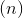are held constant; therefore, the volume and temperature are directly proportional. If the question were asking about an ideal gas, the volume would double when you double the temperature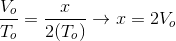The volume would double for an ideal gas; however, the question is asking about a real gas. To find the correct relationship between volume and temperature we need to look at the equation for real gas volume. Remember that the volume we are concerned with is the volume of the free space in the container, given by the container volume minus the volume of the gas particles. The equation for real gas volume accounts for the volume of the container and the volume of the gas particles. For a real gas, the volume is given as follows: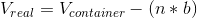In this equation,is the number of moles of gas particles andis the bigness coefficient. This equation implies that the volume of free space for a real gas is always less than the volume for an ideal gas; therefore, doubling the temperature will produce a volume that is less than the predicted volume for an ideal gas. Our answer, then, must be less than double the initial volume.

Note that for an ideal gas the bigness coefficient,, would be zero and the volume of free space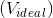would be equal to the volume of the container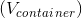. This occurs because the volume of the gas particles is negligible for an ideal gas.

### Example Question #1 : Real Gases And Ideal Gases

Which of the following is relevant for real gases, but irrelevant for ideal gases?

I. Volume of gas particles

II. Intermolecular forces between gas particles

III. Volume of container

I and III

I only

I and II

III only

I and II

Explanation:

There are two main assumptions for an ideal gas (and a few smaller assumptions). First, the gas particles of the ideal gas must have no molecular volume. Second, the gas particles must exert no intermolecular forces on each other; therefore, forces such hydrogen bonding, dipole-dipole interactions, and London dispersion forces are irrelevant in ideal gases. Other small assumptions of ideal gases include random particle motion (no currents), lack of intermolecular interaction with the container walls, and completely elastic collisions (a corollary of zero intermolecular forces).

For real gases, however, these assumptions are invalid. This means that the real gas particles have molecular volume and exert intermolecular forces on each other.

Recall that the volume in the ideal gas law is the volume of the free space available inside the container. For ideal gases, the free space volume is equal to the volume of the container because the gas particles take up no volume; however, for real gases, the free space volume is the volume of the container minus the volume of the gas particles. Though the exact values of free space volume will differ, the volume of the container is important for both real and ideal gases.

### All GRE Subject Test: Chemistry Resources